# KNN Model Complexity

KNN is a machine learning algorithm which is used for both classification (using KNearestClassifier) and Regression (using KNearestRegressor) problems.In KNN algorithm K is the Hyperparameter. Choosing the right value of K matters. A machine learning model is said to have high model complexity if the built model is having low Bias and High Variance.

We know that,

1. High Bias and Low Variance = Under-fitting model.
2. Low Bias and High Variance = Over-fitting model. [Indicated highly complex model ].
3. Low Bias and Low Variance = Best fitting model. [This is preferred ].
4. High training accuracy and Low test accuracy ( out of sample accuracy ) = High Variance = Over-fitting model = More model complexity.
5. Low training accuracy and Low test accuracy ( out of sample accuracy ) = High Bias = Under-fitting model.

Code: To understand how K value in KNN algorithm affects the model complexity.

 `# This code may not run on GFG ide ` `# As required modules are not found. ` `  `  `# Import required modules ` `import` `matplotlib.pyplot as plt ` `from` `sklearn.datasets ``import` `make_regression ` `from` `sklearn.neighbors ``import` `KNeighborsRegressor ` `from` `sklearn.model_selection ``import` `train_test_split ` `import` `numpy as np ` `  `  `# Synthetically Create Data Set ` `plt.figure() ` `plt.title(``'SIMPLE-LINEAR-REGRESSION'``) ` `x, y ``=` `make_regression( ` `    ``n_samples ``=` `100``, n_features ``=` `1``,  ` `    ``n_informative ``=` `1``, noise ``=` `15``, random_state ``=` `3``) ` `plt.scatter(x, y, color ``=``'red'``, marker ``=``'o'``, s ``=` `30``) ` `  `  `# Train the model. ` `knn ``=` `KNeighborsRegressor(n_neighbors ``=` `7``) ` `x_train, x_test, y_train, y_test ``=` `train_test_split( ` `    ``x, y, test_size ``=` `0.2``, random_state ``=` `0``) ` `knn.fit(x_train, y_train) ` `predict ``=` `knn.predict(x_test) ` `print``(``'Test Accuracy:'``, knn.score(x_test, y_test)) ` `print``(``'Training Accuracy:'``, knn.score(x_train, y_train)) ` `  `  `# Plot The Output ` `x_new ``=` `np.linspace(``-``3``, ``2``, ``100``).reshape(``100``, ``1``) ` `predict_new ``=` `knn.predict(x_new) ` `plt.plot( ` `    ``x_new, predict_new, color ``=``'blue'``,  ` `    ``label ``=``"K = 7"``) ` `plt.scatter(x_train, y_train, color ``=``'red'` `) ` `plt.scatter(x_test, predict, marker ``=``'^'``, s ``=` `90``) ` `plt.legend() `

Output: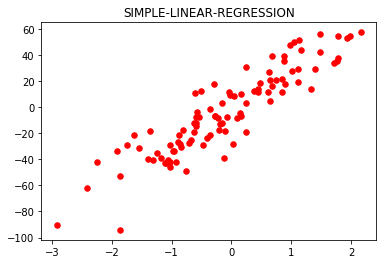```Test Accuracy: 0.6465919540035108
Training Accuracy: 0.8687977824212627
```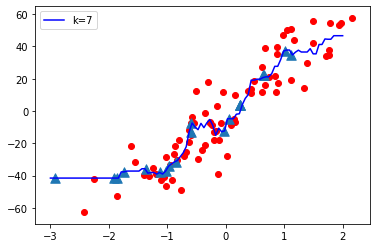Now let’s vary the value of K (Hyperparameter) from Low to High and observe the model complexity
K = 1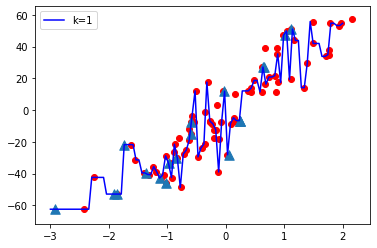K = 10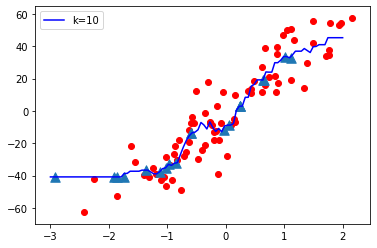K = 20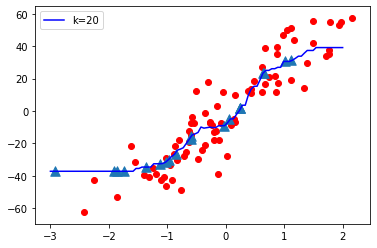K = 50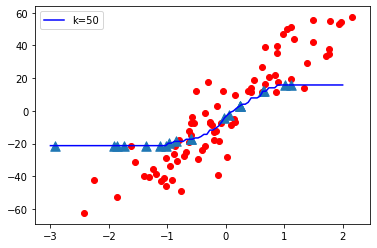K = 70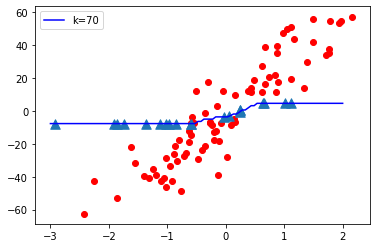Observations:

• When K value is small i.e. K=1, The model complexity is high ( Over-fitting or High Variance).
• When K value is very large i.e. K=70, The model complexity decreases ( Under-fitting or High Bias ).

Conclusion:
As K value becomes small model complexity increases and as K value becomes large the model complexity decreases.

Code: Let’s consider the below plot

 `# This code may not run on GFG ` `# As required modules are not found. ` ` `  `# To plot test accuracy and train accuracy Vs K value. ` `p ``=` `list``(``range``(``1``, ``31``)) ` `lst_test ``=``[] ` `lst_train ``=``[] ` `for` `i ``in` `p: ` `    ``knn ``=` `KNeighborsRegressor(n_neighbors ``=` `i) ` `    ``knn.fit(x_train, y_train) ` `    ``z ``=` `knn.score(x_test, y_test) ` `    ``t ``=` `knn.score(x_train, y_train) ` `    ``lst_test.append(z) ` `    ``lst_train.append(t) ` `     `  `plt.plot(p, lst_test, color ``=``'red'``, label ``=``'Test Accuracy'``) ` `plt.plot(p, lst_train, color ``=``'b'``, label ``=``'Train Accuracy'``) ` `plt.xlabel(``'K VALUES --->'``) ` `plt.title(``'FINDING BEST VALUE FOR K'``) ` `plt.legend() `

Output: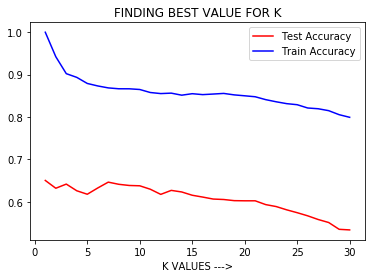Observation:
From the above graph, we can conclude that when K is small i.e. K=1, Training Accuracy is High but Test Accuracy is Low which means the model is over-fitting ( High Variance or High Model Complexity). When the value of K is large i.e. K=50, Training Accuracy is Low as well as Test Accuracy is Low which means the model is under-fitting ( High Bias or Low Model Complexity ).

So Hyperparameter tuning is necessary i.e. to select the best value of K in KNN algorithm for which the model has Low Bias and Low Variance and results in a good model with high out of sample accuracy.

We can use GridSearchCV or RandomSearchCv to find the best value of hyper parameter K.

Attention geek! Strengthen your foundations with the Python Programming Foundation Course and learn the basics.

To begin with, your interview preparations Enhance your Data Structures concepts with the Python DS Course.

My Personal Notes arrow_drop_upCheck out this Author's contributed articles.

If you like GeeksforGeeks and would like to contribute, you can also write an article using contribute.geeksforgeeks.org or mail your article to contribute@geeksforgeeks.org. See your article appearing on the GeeksforGeeks main page and help other Geeks.

Please Improve this article if you find anything incorrect by clicking on the "Improve Article" button below.